# Computer Networks (CS425)

### Instructor: Dr. Dheeraj Sanghi

Prev| Next| Index

## Data Encoding

### Digital data to analog signals

A modem (modulator-demodulator) converts digital data to analog signal. There are 3 ways to modulate a digital signal on an analog carrier signal.
1. Amplitude shift keying (ASK): is a form of modulation which represents digital data as variations in the amplitude of a carrier wave. Two different amplitudes of carrier frequency represent '0' , '1'.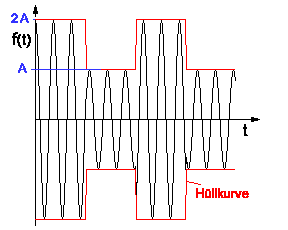2. Frequency shift keying (FSK): In Frequency Shift Keying, the change in frequency define different digits. Two different frequencies near carrier frequency represent '0' ,''1'.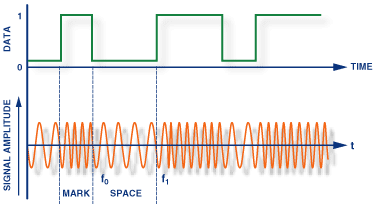3. Phase shift keying (PSK): The phase of the carrier is discretely varied in relation either to a reference phase or to the phase of the immediately preceding signal element, in accordance with data being transmitted. Phase of carrier signal is shifted to represent '0' , '1'.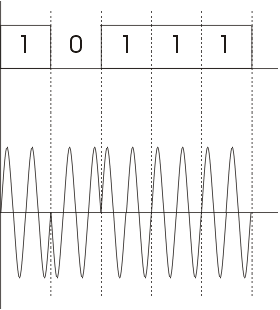### Digital data to digital signals

A digital signal is sequence of discrete , discontinuous voltage pulses. Each pulses a signal element. Encoding scheme is an important factor in how successfully the receiver interprets the incoming signal.

### Encoding Techniques

Following are several ways to map data bits to signal elements.
• Non return to zero(NRZ) NRZ codes share the property that voltage level is constant during a bit interval. High level voltage = bit 1 and Low level voltage = bit 0. A problem arises when there is a long sequence of 0s or 1s and the volatage level is maintained at the same value for a long time. This creates a problem on the recieving end because now, the clock synchronization is lost due to lack of any transitions and hence, it is difficult to determine the exact number of 0s or 1s in this sequence.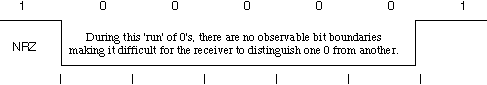The two variations are as follows:
1. NRZ-Level: In NRZ-L encoding, the polarity of the signal changes only when the incoming signal changes from a 1 to a 0 or from a 0 to a 1. NRZ-L method looks just like the NRZ method, except for the first input one data bit. This is because NRZ does not consider the first data bit to be a polarity change, where NRZ-L does.
2. NRZ-Inverted: Transition at the beginning of bit interval = bit 1 and No Transition at beginning of bit interval = bit 0 or vicecersa. This technique is known as differential encoding.

NRZ-I has an advantage over NRZ-L. Consider the situation when two data wires are wrongly connected in each other's place.In NRZ-L all bit sequences will get reversed (B'coz voltage levels get swapped).Whereas in NAZ-I since bits are recognized by transition the bits will be correctly interpreted. A disadvantage in NRZ codes is that a string of 0's or 1's will prevent synchronization of transmitter clock with receiver clock and a separate clock line need to be provided.

• Biphase encoding: It has following characteristics:
1. Modulation rate twice that of NRZ and bandwidth correspondingly greater. (Modulation is the rate at which signal level is changed).
2. Because there is predictable transition during each bit time,the receiver can synchronize on that transition i.e. clock is extracted from the signal itself.
3. Since there can be transition at the beginning as well as in the middle of the bit interval the clock operates at twice the data transfer rate.
Types of Encoding -->
• Biphase-manchester: Transition from high to low in middle of interval = 1 and Transition from low to high in middle of interval = 0
• Differential-manchester: Always a transition in middle of interval. No transition at beginning of interval=1 and Transition at beginning of interval = 0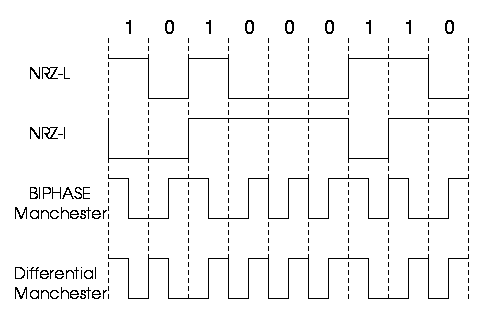• 4B/5B Encoding: In Manchester encoding scheme , there is a transition after every bit. It means that we must have clocks with double the speed to send same amount of data as in NRZ encodings. In other words, we may say that only 50% of the data is sent. This performance factor can be significantly improved if we use a better encoding scheme. This scheme may have a transition after fixed number of bits instead of every other bit. Like if we have a transition after every four bits, then we will be sending 80% data of actual capacity. This is a significant improvement in the performance.

This scheme is known as 4B/5B. So here we convert 4-bits to 5-bits, ensuring at least one transition in them. The basic idea here is that 5-bit code selected must have :

• no more than two trailing 0s
Thus it is ensured that we can never have more than three consecutive 0s. Now these 5-bit codes are transmitted using NRZI coding thus problem of consecutive 1s is solved.

The exact transformation is as follows :

4-bit Data 5-bit code4-bit Data5-bit code
000011110100010010
000101001100110011
001010100101010110
001110101101110111
010001010110011010
010101011110111011
011001110111011100
011101111111111101

Of the remaining 16 codes, 7 are invalid and others are used to send some control information like line idle(11111), line dead(00000), Halt(00100) etc.

There are other variants for this scheme viz. 5B/6B, 8B/10B etc. These have self suggesting names.

• 8B/6T Encoding: In the above schemes, we have used two/three voltage levels for a signal. But we may altogether use more than three voltage levels so that more than one-bit could be send over a single signal. Like if we use six voltage levels and we use 8-bits then the scheme is called 8B/6T. Clearly here we have 729(3^6) combinations for signal and 256(2^8) combinations for bits.
• Bipolar AIM: Here we have 3 voltage levels: middle,upper,lower
• Representation 1: Middle level =0 Upper,Lower level =1 such that successive 1's will be represented alternately on upper and lower levels.
• Representation 2 (pseudoternary): Middle level =1 Upper,Lower level=0

### Analog data to digital signal:

The process is called digitization. Sampling frequency must be at least twice that of highest frequency present in the the signal so that it may be fairly regenerated. Quantization - Max. and Min values of amplitude in the sample are noted. Depending on number of bits (say n) we use we divide the interval (min,max) into 2(^n) number of levels. The amplitude is then approximated to the nearest level by a 'n' bit integer. The digital signal thus consists of blocks of n bits.On reception the process is reversed to produce analog signal. But a lot of data can be lost if fewer bits are used or sampling frequency not so high.
• Pulse code modulation(PCM): Here intervals are equally spaced. 8 bit PCB uses 256 different levels of amplitude. In non-linear encoding levels may be unequally spaced.
• Delta Modulation(DM): Since successive samples do not differ very much we send the differences between previous and present sample. It requires fewer bits than in PCM.

### Digital Data Communication Techniques:

For two devices linked by a transmission medium to exchange data ,a high degree of co-operation is required. Typically data is transmitted one bit at a time. The timing (rate, duration,spacing) of these bits must be same for transmitter and receiver. There are two options for transmission of bits.
1. Parallel All bits of a byte are transferred simultaneously on separate parallel wires. Synchronization between multiple bits is required which becomes difficult over large distance. Gives large band width but expensive. Practical only for devices close to each other.
2. Serial Bits transferred serially one after other.Gives less bandwidth but cheaper. Suitable for transmission over long distances.

Transmission Techniques:

1. Asynchronous: Small blocks of bits(generally bytes) are sent at a time without any time relation between consecutive bytes .when no transmission occurs a default state is maintained corresponding to bit 1. Due to arbitrary delay between consecutive bytes,the time occurrences of the clock pulses at the receiving end need to be synchronized for each byte. This is achieved by providing 2 extra bits start and stop.

Start bit: It is prefixed to each byte and equals 0. Thus it ensures a transition from 1 to 0 at onset of transmission of byte.The leading edge of start bit is used as a reference for generating clock pulses at required sampling instants. Thus each onset of a byte results in resynchronization of receiver clock.

Stop bit: To ensure that transition from 1 to 0 is always present at beginning of a byte it is necessary that default state be 1. But there may be two bytes one immediately following the other and if last bit of first byte is 0, transition from 1 to 0 will not occur . Therefore a stop bit is suffixed to each byte equaling 1. It's duration is usually 1,1.5,2 bits.

Asynchronous transmission is simple and cheap but requires an overhead of 3 bits i.e. for 7 bit code 2 (start ,stop bits)+1 parity bit implying 30% overhead.However % can be reduced by sending larger blocks of data but then timing errors between receiver and sender can not be tolerated beyond [50/no. of bits in block] % (assuming sampling is done at middle of bit interval). It will not only result in incorrect sampling but also misaligned bit count i.e. a data bit can be mistaken for stop bit if receiver's clock is faster.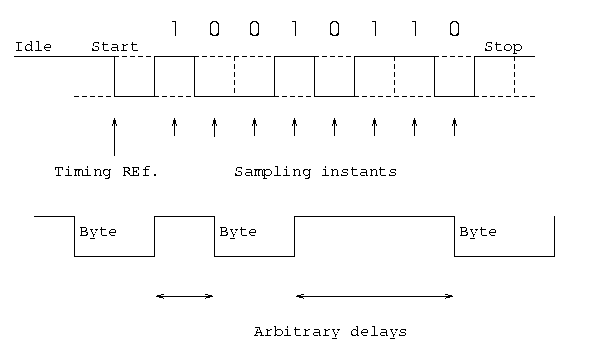2. Synchronous - Larger blocks of bits are successfully transmitted.Blocks of data are either treated as sequence of bits or bytes. To prevent timing drift clocks at two ends need to be synchronized.This can done in two ways:
1. Provide a separate clock line between receiver and transmitter. OR
2. Clocking information is embedded in data signal i.e. biphase coding for digital signals.
Still another level of synchronization is required so that receiver determines beginning or end of block of data. Hence each block begins with a start code and ends with a stop code.These are in general same known as flag that is unique sequence of fixed no. of bits.In addition some control characters encompass data within these flags. Data+control information is called a frame. Since any arbitrary bit pattern can be transmitted there is no assurance that bit pattern for flag will not appear inside the frame thus destroying frame level synchronization. So to avoid this we use bit stuffing

Bit Stuffing: Suppose our flag bits are 01111110 (six 1's). So the transmitter will always insert an extra 0 bit after each occurrence of five 1's (except for flags). After detecting a starting flag the receiver monitors the bit stream . If pattern of five 1's appear, the sixth is examined and if it is 0 it isdeleted else if it is 1 and next is 0 the combination is accepted as a flag. Similarly byte stuffing is used for byte oriented transmission.Here we use an escape sequence to prefix a byte similar to flag and 2 escape sequences if byte is itself a escape sequence.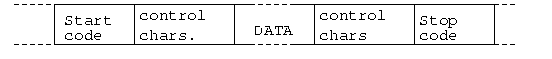### Image References:

• http://www.analog.com/library/analogDialogue/archives/38-08/DDS_06.gif
• http://racon.net/archive/FHTW/projects/Richtfunk/2psk.png
• http://fara.cs.uni-potsdam.de/~rnitschk/wireless/antenna/pics/009001.gif
• http://www.erg.abdn.ac.uk/users/gorry/course/images/nrz-run.gif